# Problem 49733. Solve the arithmetic differential equation D(n) = n

Cody Problem 47843 involved the arithmetic derivative of integers. In particular,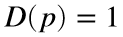if p is prime and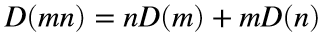. Therefore, the arithmetic derivatives of 1, 2, 3, 4, 5, and 6 are 0, 1, 1, 4, 1, and 5, respectively.
One might then ask about solving arithmetic differential equations (ADEs). Because the study of differential equations often starts with solving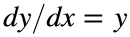, let’s consider the analogous ADE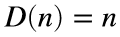. The definition of the arithmetic derivative shows that no prime can solve this equation, but the sample calculations above show that the first (i.e.,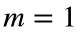) solution is 4.
Write a function to compute the mth solution to this ADE. Because the solutions become large quickly, return the logarithm of the solution.

### Solution Stats

47.83% Correct | 52.17% Incorrect
Last Solution submitted on Jun 17, 2023

### Community Treasure Hunt

Find the treasures in MATLAB Central and discover how the community can help you!

Start Hunting!